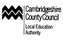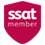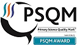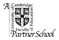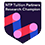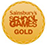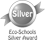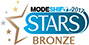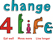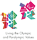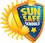Mathematics

What does Mathematics look like at Meldreth Primary?
Our mathematics curriculum provides a foundation for understanding the world, the ability to reason mathematically, an appreciation of the beauty and power of mathematics, and a sense of enjoyment and curiosity about the subject.

Mathematics is taught in a meaningful and enjoyable way that links to the world around us and develops an enquiry approached curriculum. Within school children are taught in mixed ability groups through a Mastery Approach. Our curriculum is designed systematically to build, extend and challenge the skills, knowledge and understanding that children have.

Within our school there is a dedicated time to ‘basic skills’ teaching outside of the daily maths lesson. Children work in 'Magic Ten' sessions each day where the focus is upon the key principles of children being ‘numerate’.

Our Curriculum Aims
Our curriculum for mathematics aims to ensure that all pupils:
• become fluent in the fundamentals of mathematics, including through varied and frequent practice with increasingly complex problems over time, so that pupils develop conceptual understanding and the ability to recall and apply knowledge rapidly and accurately
• reason mathematically by following a line of enquiry, conjecturing relationships and generalisations, and developing an argument, justification or proof using mathematical language
• can solve problems by applying their mathematics to a variety of routine and non-routine problems with increasing sophistication, including breaking down problems into a series of simpler steps and persevering in seeking solutions
Additionally, our curriculum encourages pupils to talk about their mathematical reasoning, which is a key factor in developing mathematical vocabulary and presenting a mathematical justification, argument or proof. In this way, pupils learn to make their thinking clear to themselves as well as others, and teachers ensure that pupils build secure foundations by using discussion to probe and remedy any misconceptions.

Curriculum Content
Key Stage 1 (Years 1 and 2)
The principal focus of our mathematics teaching in Key Stage 1 is to ensure that pupils develop confidence and mental fluency with whole numbers, counting and place value. This involves working with numerals, words and the four operations; children will have the opportunities to use practical resources (for example, concrete objects and measuring tools) to embed their learning. At this stage, pupils develop their ability to recognise, describe, draw, compare and sort different shapes and use the related vocabulary. Teachers also help pupils to use a range of measures to describe and compare different quantities such as length, mass, capacity / volume, time and money. By the end of Year 2, pupils will know the number bonds to 20 and be precise in using and understanding place value. An emphasis is placed on practice at this early stage. The curriculum ensures that pupils are able to read and spell mathematical vocabulary, at a level consistent with their increasing word-reading and spelling knowledge at Key Stage 1. Over the course of the two years pupils will cover:

Lower Key Stage 2 (Year 3 and 4)
The principal focus of our mathematics curriculum in lower Key Stage 2 is to ensure that pupils become increasingly fluent with whole numbers and the four operations, including number facts and the concept of place value. This ensures that pupils develop efficient written and mental methods and perform calculations accurately with increasingly large whole numbers. We aim to also make sure that pupils also develop their ability to solve a range of problems, including simple fractions and decimal place value. Teaching also ensures that pupils draw with increasing accuracy and develop mathematical reasoning so they can analyse shapes and their properties, and confidently describe the relationships between them. Our curriculum ensures that they can use measuring instruments with accuracy and make connections between measure and number. By the end of Year 4, it is our aim that pupils will have memorised their multiplication tables up to and including the 12 multiplication table and show precision and fluency in their work. They should be able to read and spell mathematical vocabulary correctly and confidently, using their growing sight vocabulary and knowledge of spelling.

Upper Key Stage 2 (Years 5 and 6)
The principal focus of our mathematics curriculum in upper Key Stage 2 is to ensure that pupils extend their understanding of the number system and place value to include larger integers. This should develop the connections that pupils make between multiplication and division with fractions, decimals, percentages and ratio. At this stage, pupils develop their ability to solve a wide range of problems, including increasingly complex properties of numbers and arithmetic, and problems demanding efficient written and mental methods of calculation. With this foundation in arithmetic, pupils are introduced to the language of algebra as a means for solving a variety of problems. Teaching in geometry and measures aims to consolidate and extend knowledge developed in number. Teaching also ensures that pupils classify shapes with increasingly complex geometric properties and pupils learn the vocabulary they need to describe them. By the end of Year 6, the curriculum followed aims to make sure that pupils are fluent in written methods for all four operations, including long multiplication and division, and in working with fractions, decimals and percentages. Pupils are able to read, spell and pronounce increasingly complex mathematical vocabulary correctly.

Over the course of the curriculum, all pupils will learn about:
• Number - number and place value
• Number - addition and subtraction
• Number - multiplication and division
• Number - fractions
• Measurement
• Geometry - properties of shapes
• Geometry - position and direction
• Statistics

What your child will learn in Mathematics over their time with us

Progress that children should make is outlined below in each area of mathematical learning. Some aspects of mathematical learning have central relevance to more than one area of learning and will appear in each area to reflect that pupils should make rich connections across mathematical ideas to develop fluency, mathematical reasoning and competence in solving increasingly sophisticated problems.

Our Approach to  Calculation

Through our rich and progressive mathematics curriculum, at Meldreth Primary School children are taught to become fluent with key written methods for addition, subtraction, multiplication and division.

A variety of methods are used to teach children calculation methods, which include a range of concrete, pictoral and abstract methods in support all our learners. Teachers will expose children a variety of teaching methods so that children develop the skills to fluently move between representations of the calculation method. For example: missing digits, money or algebra.

As part of a child’s learning in calculation, we teach how to select the best method according to the problem being solved. The hierarchy of thinking we teach is:
• Can I do it in my head?
• Can I use some jottings to help me?
• Should I use a written method?